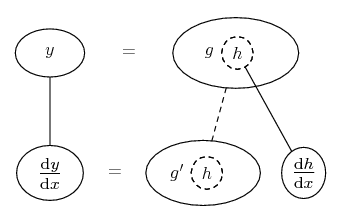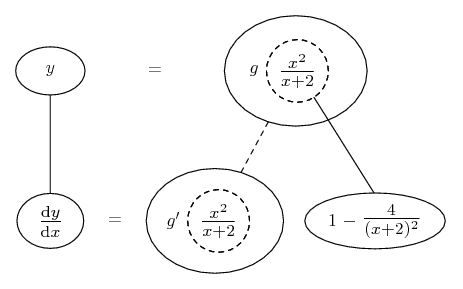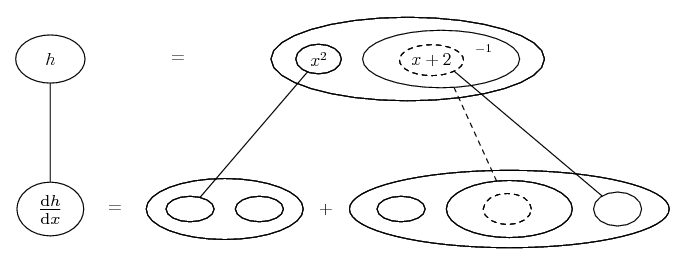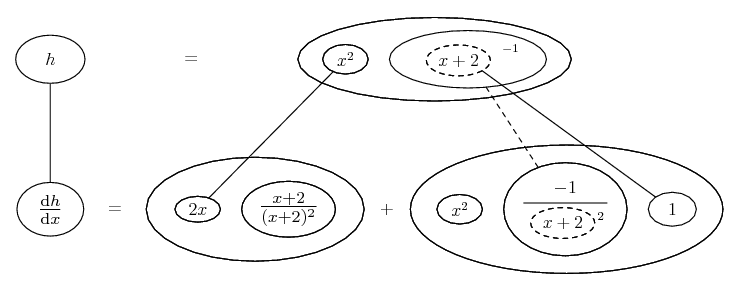1. ## Derivatives

Let y = g(h(x)) where h(x) = x^2/x+2. If g'(9/5) = -2, find dy/dx when x = 3.

2. Just in case a picture helps... We're told the top level, and can infer the lower, of...... and more specifically...... where... is the chain rule. Straight continuous lines differentiate downwards (integrate up) with respect to x, and the straight dashed line similarly but with respect to the dashed balloon expression (the inner function of the composite which is subject to the chain rule).

And plug in 3 for x (so that you can in turn use the given value for g'(9/5)), to evaluate dy/dx. How did we differentiate the inner function, though? One way is to use the chain rule wrapped in the legs-uncrossed version of...... the product rule, for...... i.e. pretty much the quotient rule.

Spoiler:Complete the square in the numerator to find you have the same square as in the denominator but minus 4. Hence: 1, minus 4 over the square.

Or you could first simplify h by long division (as it's top-heavy).
_________________________________________
Don't integrate - balloontegrate!

Balloon Calculus; standard integrals, derivatives and methods

Balloon Calculus Drawing with LaTeX and Asymptote!

#### Search Tags

derivatives